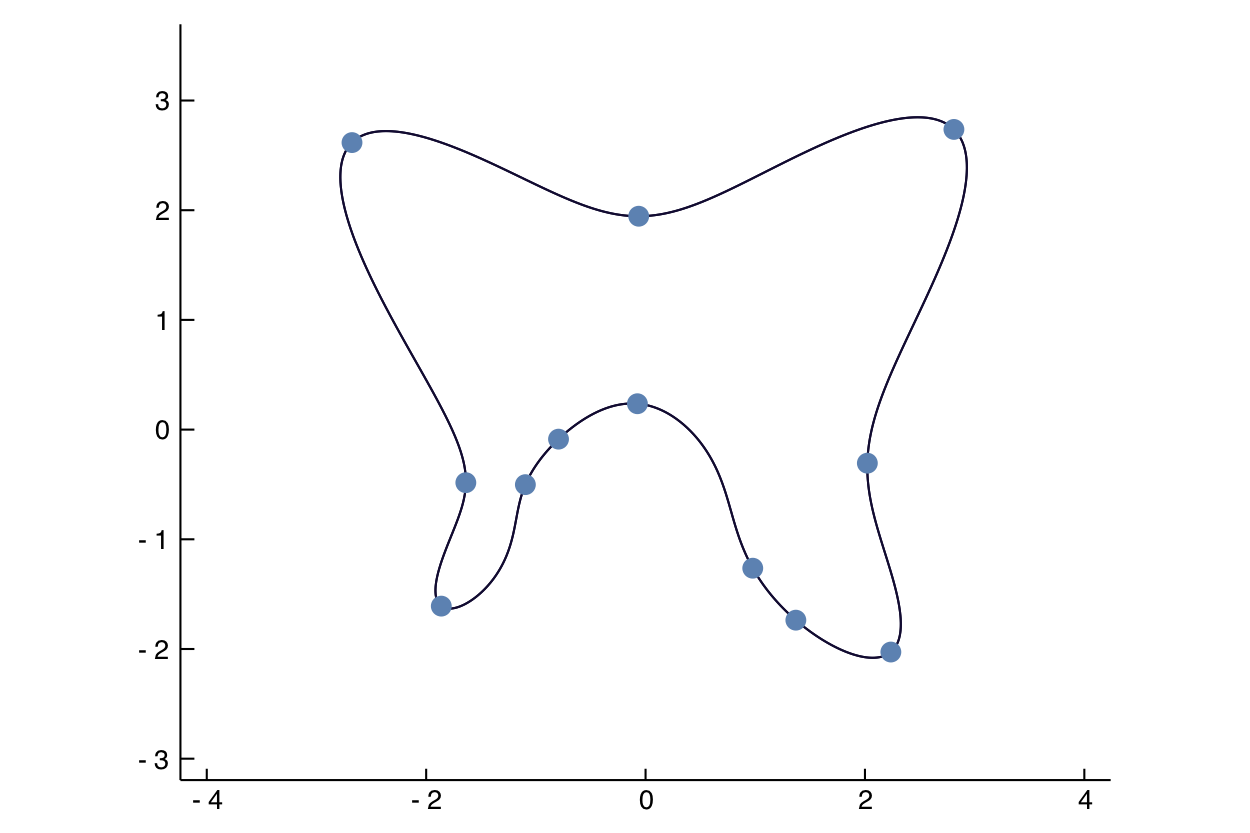# Curvature of plane curves

How to compute curvature

### Curvature of curves in the plane

Consider the problem of computing the point on a (smooth) curve $V\subset \mathbb{R}^n$, where the curvature is maximal. We can use the following formula for curvature at a point $p\in V = \{f(x_1,x_2)=0\}$.

$$\sigma(p) = \frac{v^T H v}{g^\frac{3}{2}}$$

where $v^T \nabla_p f(p) = 0$, and $v^T v=1$, and $H$ is the Hessian of $f$ at $p$, and $g = \nabla_p f(p)^T\nabla_p f(p)$. The conditions for $\sigma(p)$ being maximal on $V$ are thus $v^T \nabla_p \sigma(p)=0$ and $f(p)=0$.

Thus, for maximizing $\sigma$ over

$$V =\{x_1^4 - x_1^2x_2^2 + x_2^4 - 4x_1^2 - 2x_2^2 - x_1 - 4x_2 + 1 = 0\}$$

we use the following code.

using HomotopyContinuation, LinearAlgebra

@var x[1:2]# initialize variables
f = x^4 - x^2*x^2 + x^4 - 4x^2 - 2x^2 - x - 4x + 1

∇ = differentiate(f, x) # the gradient
H = differentiate(∇, x) # the Hessian

g = ∇ ⋅ ∇
v = [-∇; ∇]
h = v' * H * v
dg = differentiate(g, x)
dh = differentiate(h, x)

F = [(g .* dh - ((3/2) * h).* dg) ⋅ v; f]

solve(F)

Result with 56 solutions
========================
• 64 paths tracked
• 56 non-singular solutions (12 real)
• random_seed: 0xa10d5d18
• start_system: :polyhedral


Here is a picture of all solutions.Cite this example:
@Misc{ curvature2022 ,
author =  { Paul Breiding },
title = { Curvature of plane curves },
howpublished = { \url{ https://www.JuliaHomotopyContinuation.org/examples/curvature/ } },
note = { Accessed: June 27, 2022 }
}